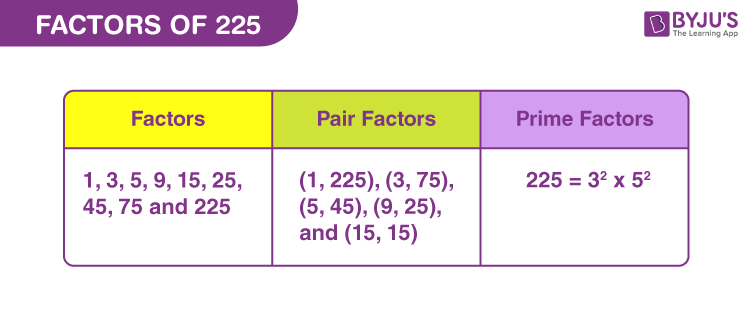# Factors of 225

Factors of 225 are the natural numbers, by which the original number is completely divisible.Thus, factors are also called the divisor of natural numbers, sometimes. If the multiplication of two natural numbers is equal to 225, they become the factors. 225 is a composite number. Also, 225 is divisible by 5 (by divisibility rules).

Since, 15 x 15 = 225 = 152

Therefore, another important property of 225 is it is a perfect square.

Let us find the factors of 225 along with pair factors and prime factors.## How to Find the Factors of 225?

To find the factors of 225, we need to check, by which numbers or integers, 225 is completely divisible. Since, we know, every number is divisible by itself and 1. Thus, the two factors are already determined. Now the rest of factors can be evaluated by the division method.

225 ÷ 1 = 225

225 ÷ 3 = 75

225 ÷ 5 = 45

225 ÷ 9 = 25

225 ÷ 15 = 15

225 ÷ 25 = 9

225 ÷ 45 = 5

225 ÷ 75 = 3

225 ÷ 225 = 1

Therefore, we can conclude that there are a total nine factors of 85, i.e., 1, 3, 5, 9, 15, 25, 45, 75 and 225.

## Pair Factors of 225

When the product of two integers results in 225, then they become the pair factors. Hence,

• 1 × 225 = 225
• 3 × 75 = 225
• 5 × 45 = 225
• 9 × 25 = 225
• 15 × 15 = 225

Therefore, the pair factors of 225 are (1, 225), (3, 75), (5, 45), (9,25) and (15, 15).

We can consider to multiply two negative numbers to get the factors in pairs, such that;

• -1 × -225 = 225
• -3 × -75 = 225
• -5 × -45 = 225
• -9 × -25 = 225
• -15 × -15 = 225

Therefore, the negative pair factors are (-1, -225), (-3, -75), (-5, -45), (-9, -25) and (-15, -15).

## Prime Factorisation of 225

Prime factorisation of 225 will produce the prime factors form of original number. Let us see, what are the prime factors of 225.

 Dividing 225 by smallest prime number 225 ÷ 3 = 75 Again dividing 75 by smallest prime number 75 ÷ 3 = 25 Dividing 25 by 5 25 ÷ 5 = 5 Again, dividing 5 by 5 5 ÷ 5 = 1

Therefore, prime factors of 225 are 3 and 5.

 Prime factorisation of 225 = 3 x 3 x 5 x 5 Exponential form = 32 x 52

## Solved Examples

Q.1: There are 45 students in a classroom. Seema wants to distribute 225 chocolates on the occasion of her birthday to all the students. How can she divide and distribute the chocolates to each student?

Solution: Given,

Number of students = 45

Number of chocolates = 225

Number of chocolates each student should get = 225/45 = 5

Therefore, Seema has to give 5 chocolates to each student.

Q.2: What is the sum of all the factors of 225?

Solution: The factors of 225 are 1, 3, 5, 9, 15, 25, 45, 75, and 225.

Sum = 1+3+5+9+15+25+45+75+225 = 403

Therefore, the required sum is 403.

Q.3: What are the common factors of 255 and 225?

Solution: Let us list the factors of both the numbers 117 and 225.

225 → 1, 3, 5, 9, 15, 25, 45, 75, 225

255 → 1, 3, 5, 15, 17, 51, 85, 255

Therefore, the common factors of 225 and 255 are 1, 3, 5 and 15.

Q.4: Find the GCF of 200 and 225.

Solution: The individual factors are:

200 → 1, 2, 4, 5, 8, 10, 20, 25, 40, 50, 100, 200

225 → 1, 3, 5, 9, 15, 25, 45, 75, 225

Therefore, the greatest common factor of 200 and 225 is:

GCF (200, 225) = 25

### Practice Questions

1. Find the average of factors of 225.
2. Find the common factors of 225 and 25.
3. Is 20 a factor of 225?
4. What are the natural numbers that divide 225, evenly?
5. Which of these is not a factor of 225?
1. 25
2. 15
3. 55
4. 75

## Frequently Asked Questions on Factors of 225

### What are the factors of 225?

The factors of 225 are 1, 3, 5, 9, 15, 25, 45, 75, and 225.

### What are the prime factors of 225?

225 is divisible by only two prime factors, i.e. 3 and 5.

### What is the 10th multiple of 225?

225 multiplied by 10 is equal to 2250. Therefore, the tenth multiple is 2250.

### Which is the highest factor of 225?

The highest factor of 225 is 225 itself and the second highest factor is 75.

### Is 225 a perfect square?

225 is a perfect square because the square root of 225 will result in a whole number.
square root of 225, √225 = 15.

### What is GCF of 180 and 225?

The factors of 180 is 1, 2, 3, 4, 5, 6, 9, 10, 12, 15, 18, 20, 30, 36, 45, 60, 90, 180 and factors of 225 is 1, 3, 5, 9, 15, 25, 45, 75, 225. Thus we can see, 45 is the greatest common factor of 180 and 225.

### What is the GCF of 225 and 36?

GCF of 225 and 36 is 9.